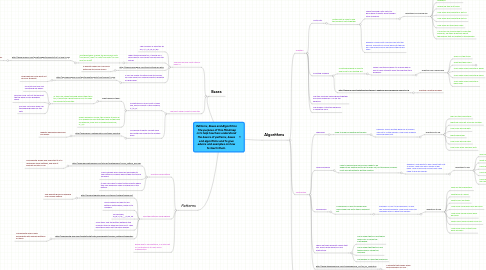Patterns, Bases and Algorithms The purpose of this Mindmap is to help teachers understand the ba...

Just an initial demo map, so that you don't start with an empty map list ...

Get Started. It's FreePatterns, Bases and Algorithms The purpose of this Mindmap is to help teachers understand the basics of patterns, bases and algorithms and to give advice and examples on how to teach them.1. Patterns

1.1. Find the visual pattern

1.1.1. http://www.perceptualedge.com/articles/Whitepapers/Visual_Pattern_Rec.pdf

1.1.1.1. This website shows how important it is to recognize visual patterns, and how it benefits us later in life

1.1.2. Many children learn easier by being able to see patterns in shapes before they start using numbers.

1.1.3. It may even help to have tactile objects where they can physically make a difference in the pattern.

1.2. Find the patterns using algebra

1.2.1. http://www.integretechpub.com/gallery/mathex/sequence/

1.2.1.1. This website gives an example of a number pattern.

1.2.2. Once children are able to see patterns with shapes, move on to numbers.

1.2.3. For example, 3,6,9,12,15,__,__,__,27,33, 36

1.2.4. Have them find the pattern between the numbers they do know and discuss it. Then have them figure out the blank spaces.

1.2.5. http://cybersleuth-kids.com/sleuth/Math/Math_Worksheets/Number_Patterns/index.html

1.2.5.1. This website offers many worksheets with number patterns on them.

2. Algorithms

2.1.1. Unite Sets

2.1.1.1. Uniting sets is used to add two different sets together.

2.1.1.1.1. When teaching Unite Sets it is good have students work through story problems.

2.1.1.1.2. Example: Tommy put 3 soccer balls into the ball bin. Alex put for soccer balls into the ball bin. How many soccer balls are in the ball bin now?

2.1.2. Counting Forward

2.1.2.1. Counting forward is used to add more to an existing set.

2.1.2.1.1. When Counting forward it is a good idea as well to have students work through the story problems.

2.1.2.2.1. Practice counting forward.

2.1.3. The two numbers being added together are called Addends. 2+2 are the addends

2.1.4. The answer of the two addends is called the Sum.

2.2. Subtraction

2.2.1. Take away

2.2.1.1. Used to make an existing set smaller.

2.2.1.1.1. Example: Alice's brother gave her 6 markers. She lost 4 of the markers. How many markers does she have left?

2.2.2.1. used to decide how much more needs to be added to an existing set to get a larger set, but the given numbers must be subtracted to get the solution.

2.2.2.1.1. Example: Jane wants to buy candy that cost 3 dollars. Jane only has 2 dollars in her bank. How much more money does Jane need to buy her candy?

2.2.3. Comparison

2.2.3.1. Comparison is used to decide how much larger one set is than a different set.

2.2.3.1.1. Example: AJ has 10 red airplanes. Aj also has 5 yellow airplanes. How many more red airplanes does AJ have than yellow?

2.2.4. There are three different names that you should know when you are subtracting.

2.2.4.1. The number that you are taking away from is called the subtrahend.

2.2.4.2. The number that that you are taking away is called the minuend.

2.2.4.3. The answer is called the difference

2.2.5. http://www.thinkingblocks.com/ThinkingBlocks_AS/TB_AS_Main.html

2.2.5.1. A website that breaks down word problems for you.

2.3.1. Website that allows you to work on word problems with addition, subtraction, multiplication and division

3. Bases

3.1. The base we use most often is base 10.

3.1.1. The numbers in base ten go are, 0,1,2,3,4,5,6,7,8,9

3.1.2. When teaching base ten, it would be a good idea to use visuals such as base ten blocks.

3.1.2.1. The three types of base ten blocks are units (one block), rods (10 units) and flats (10 rods and 100 units)

3.1.2.1.1. http://www.enasco.com/prod/images/products/F3/VC132617l.jpg

3.1.3. http://www.learningbox.com/Base10/BaseTen.html

3.1.3.1. A website where you can work with base ten block online!

3.1.4. If you are unable to obtain base ten blocks, you may have your children practice drawing on grid paper.

3.1.4.1. http://wps.ablongman.com/wps/media/objects/778/796754/17.pdf

3.1.4.1.1. Grid paper you may print out for your students

3.2. We don't always count in base ten.

3.2.1. Sometimes you may count in base five, which consist of the numbers, 0,1,2,3,4

3.2.1.1. What happens then?

3.2.1.1.1. In this case, when teaching bases other than ten, it would be a great idea for you to make the visuals to teach this.

3.2.1.2. When working in a base, the number it goes up to is always one less than the name of the base. For example, base four's numbers would be, 0,1,2,3

3.2.2. Try having students convert back and forth from base ten to another base.

3.2.2.1. http://www.basic-mathematics.com/base-five.html

3.2.2.1.1. Website explaining how base five works.